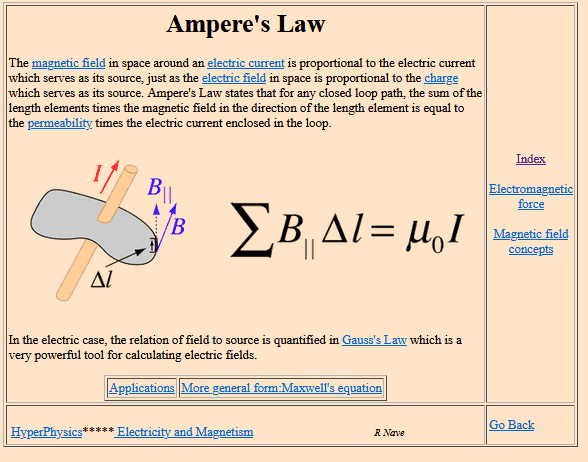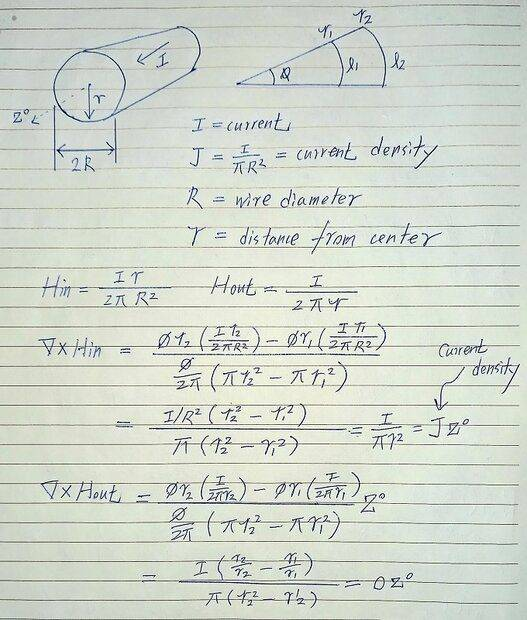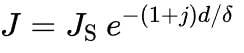Current vs Current Density vs Magnetic Field

Summary:
If I want to decrease the magnetic field a certain distance away from a point, increasing the conductor to reduce current density help?
If I want to carry 10 A through an 8 AWG wire, I understand that there will be a specific magnetic field around the wire which will decrease as I move outward from the wire radially. Let's consider the center of that wire as coordinates (x,y)=(0,0). An object located at (0,10) would see a certain magnetic field from the current in the wire. If I use a thicker wire (lets say 4 AWG) and place the center at the same (0,0), the conductor itself will be physically closer to the object at (0,10) but what would be the effect on the magnetic field?

berkeman
Mentor
Are you familiar with Ampere's Law? If the same current is enclosed by the same loop (at your specified radius), then the B-field is the same:http://hyperphysics.phy-astr.gsu.edu/hbase/magnetic/amplaw.html

Can you say more about what you want to shield? There can be other options to accomplish what you need...

•dlgoff
Are you familiar with Ampere's Law? If the same current is enclosed by the same loop (at your specified radius), then the B-field is the same:

View attachment 295403
http://hyperphysics.phy-astr.gsu.edu/hbase/magnetic/amplaw.html

Can you say more about what you want to shield? There can be other options to accomplish what you need...
Hi Berkeman,

Thanks for your response. I'm somewhat familiar with Ampere's law, but the equation shown is simplified. Going to Maxwell's equations, it appears that the curl of H is related to current density. So I was thinking that reducing current density could also be an effective way to reduce magnitude of the magnetic field.

I'm not trying to shield anything necessarily. I'm just looking for simple ways to reduce the magnitude of the magnetic field by doing something like increasing the conductor size.

DaveE
Gold Member
So I was thinking that reducing current density could also be an effective way to reduce magnitude of the magnetic field.
But you haven't reduced the total current in the wire. The contribution from a small area with less current density will be smaller, but you will also have to add up all of the contributions from all of the small areas. One way to think of this is that each moving charge contributes to the total H field, you have to add them all up to get the field value. The fact that you've spread them out a bit isn't very significant.

Still, I suppose if you move the currents in space (like a bigger wire) it will have some effect on the field nearby.

berkeman
Mentor
•dlgoff
The differential form is a function of current density though.

berkeman
Mentor
Plea
The differential form is a function of current density though.
Can you please show how this helps your argument that increasing the diameter of a wire out to R that is less than the r of your measurement radius changes anything? Thank you.

berkeman
Mentor
If the current density decreases, doesn't H also decrease?
Of course. The current density enclosed by your radius measurement loop. How does that density change once you are outside of the raduis of the wire?

Baluncore
2021 Award
Summary:: If I want to decrease the magnetic field a certain distance away from a point, increasing the conductor to reduce current density help?

If I want to carry 10 A through an 8 AWG wire, I understand that there will be a specific magnetic field around the wire which will decrease as I move outward from the wire radially.
There are two easy ways to null the magnetic field due to the current.

Split the single conductor into two parallel conductors having identical resistance, then run one on each side of the required null plane. If the branch currents are the same, then the B fields half-way between the wires will cancel.

The general solution is to run the return circuit current back along a line close to the supply conductor. Twist the two circuit wires together, or for best results run them in a steel conduit, or use a coaxial cable.

I'm not trying to shield anything necessarily. I'm just looking for simple ways to reduce the magnitude of the magnetic field by doing something like increasing the conductor size.
The differential form is a function of current density though
You can of course use Ampere's law in differential form. When the current density is lower, the curl of the magnetic field is also lower. However, lower curl does not necessarily mean lower magnetic field. Obviously, if you increase the diameter of the wire, for the same low frequency current, you get a lower current density, the magnetic field inside the wire, especially at the center, may decrease, but in some places in space, such as the space outside the wire, the current density is zero, so the curl of magnetic field is also zero , but the magnetic field can still be very large.

That is, if you increase the diameter of the wire to reduce the current density, it will do nothing to reduce the magnetic field in the space outside the wire at the same distance from the origin. But, on the other hand, if you don't mind the higher price and larger wire volume, you can of course increase the conductor size to reduce copper losses.Last edited:
•DaveE
Maybe some people are interested to take a look at the details.

If the current density inside the wire is assumed to be uniform, then the curl inside the wire is the same everywhere, but the magnetic field inside the wire increases linearly with ##r## from the center point until it reaches the surface of the wire. On the other hand, the external magnetic field as a function of ##r'## is not affected by the wire diameter ##R##.

http://hyperphysics.phy-astr.gsu.edu/hbase/magnetic/magin.html

•DaveE
It is easy to show that the curl of magnetic field everywhere inside the wire is equal to the current density, and the curl of magnetic field everywhere outside the wire is zero, no calculus required.Correction : R = Wire Radius

Last edited:
•dlgoff
Maybe some people are interested to take a look at the details.

If the current density inside the wire is assumed to be uniform, then the curl inside the wire is the same everywhere, but the magnetic field inside the wire increases linearly with ##r## from the center point until it reaches the surface of the wire. On the other hand, the external magnetic field as a function of ##r'## is not affected by the wire diameter ##R##.

http://hyperphysics.phy-astr.gsu.edu/hbase/magnetic/magin.html
Thanks for the analysis. After looking at that more, one thing pops out to me. One of the assumptions here is that there is a uniform current. By making that assumption, current density J can be simplified to current I. At higher frequency, the skin effect can cause a significantly higher current density near the surface. Based on that, does that also mean it increases the magnetic field at a given distance from the conductor since the magnetic field at the surface is higher? I know magnetic field strength is also a function of frequency so I'm not referring to that, just strictly the increase in current density at the surface due to the skin effect.

Thanks for the analysis. After looking at that more, one thing pops out to me. One of the assumptions here is that there is a uniform current. By making that assumption, current density J can be simplified to current I. At higher frequency, the skin effect can cause a significantly higher current density near the surface. Based on that, does that also mean it increases the magnetic field at a given distance from the conductor since the magnetic field at the surface is higher? I know magnetic field strength is also a function of frequency so I'm not referring to that, just strictly the increase in current density at the surface due to the skin effect.

As mentioned earlier, the magnetic field outside the wire is only related to the total current inside the wire, that is, even if all the current is concentrated on the surface of the wire, it will not affect the strength of the external magnetic field of the wire.

As for the distribution of the magnetic field generated by the high-frequency current inside the wire, it is quite complicated. Even if we assume that the diameter of the wire is much larger than the skin depth, we can apply a relatively simple equation for current density as follows:Note, however, that the phase angle of this current density also varies with depth.

When the skin depth is not small relative to the wire radius, we need to use the Bessel function to describe the current density.

If you want to compare the magnetic field generated by high frequency and DC inside the wire in detail, and don't mind the complicated calculation process, maybe you can try to calculate it yourself based on the current density distribution.https://en.wikipedia.org/wiki/Skin_effect

Last edited: